Pictures by Chuttersnap and Nana Smirnova, Unsplash.

As everyone who works in ecommerce will know, stock-outs on your key lines can have a massive negative impact on sales and your marketing costs. In many cases, you’ll be paying a pound or so to get every visitor onto your site via your paid search ads, so if they land on your product page and the product they want is out of stock, you’ll be burning money.

Most of the customers who land on a product page and find a product out of stock will bounce and go back to Google to find another retailer who has what they want available to buy right now.

While some stock-outs are always inevitable and unavoidable, there are several ways procurement managers can keep their stock under tighter control to help reduce the likelihood of expensive stock-outs on the lines that contribute the most or are hardest to control.

#### Inventory management in ecommerce

ABC inventory management is the classic approach to managing stock. This calculates the revenue generated by each SKU and ranks its cumulative percentage contribution to the total revenue generated from all SKUs.

As it’s based on the Pareto principle, or 80/20 rule, the products generating the top 80% of your revenue are assigned to Class A and get close observation to prevent stock-outs because they generate so much. The next 10% of revenue-generating SKUs get assigned to Class B and get slightly less control, while the ones in Class C don’t generate much and can be spared the granular level of control given to the As and Bs.

While ABC inventory management is extremely effective, there’s more to controlling stock than simply having the most popular lines in stock to sell.

Sometimes demand for items can change over time, is seasonal, or is subject to changes in demand caused by product lifecycle, economic factors, competitor activity, or marketing promotions. These drivers all impact the ability to forecast demand for the affected SKUs, making costly stock-outs much more likely.

This is where XYZ inventory management comes in. Unlike ABC inventory management, which looks at the revenue contribution of each SKU, XYZ inventory management classifies SKUs according to the variability of their demand. When demand for products is fairly constant forecasting is relatively straightforward. However, when it’s subject to variation demand forecasting becomes a challenge - or a nightmare.

#### How XYZ inventory management works

With XYZ inventory management, the sales demand for each SKU is measured over a long period of time, in order to capture seasonal variations in demand, then the variance is calculated for each SKU and scores are ranked according to their variability.

• Class X: Products assigned to Class X are those which show the least variation in demand. Sales of these products are fairly constant, which means they’re easier for procurement managers to forecast and stock outs should be straightforward to avoid.

• Class Y: Products assigned to Class Y are more variable than those in Class X. Demand can change over time, due to factors like seasonality, so they’re harder to accurately forecast.

• Class Z: Finally, Class Z products are highly variable. Demand goes up and down and is really hard to predict, so avoid stock outs can be really challenging, unless you sit on large stocks that may not turnover quickly.

#### Combining ABC and XYZ

Since both ABC inventory management and XYZ inventory management both tackle different things and are both extremely useful to operations managers or procurement staff, they’re often combined as ABC-XYZ inventory management.

Here, as the name suggests, the ABC class and the XYZ class assignation for each SKU is concatenated to form AX, AY, AZ, BX, BY, BZ, CX, CY, and CZ classes that help managers understand both the importance to revenue and the variation in demand.

In this project we’re going to use a 12 month snapshot of ecommerce transactional data to create an ABC-XYZ inventory management model which classifies each SKU, and proposes some recommendations for their management to help the retailer avoid too many costly stock-outs.For this project we’re mostly using Pandas, plus the timedelta module from the Python datetime package, as well as Numpy for some mathematical calculations and Seaborn and Matplotlib for visualising the data. You can do this whole project in steps inside a Jupyter notebook if you want to follow along.

``````from datetime import timedelta
import pandas as pd
import numpy as np
import seaborn as sns
import matplotlib.pyplot as plt
``````
``````sns.set_style("dark")
sns.set()
``````

Since we need to know the quantity and revenue each SKU generates over time, we need transaction item data which you should be able to extract easily from your SQL database. I’ve pulled in the whole lot, but we actually only need data for the past 12 full months.

``````df_order_items = pd.read_csv('order_items_processed.csv', low_memory=False)
df_order_items = df_order_items.drop(columns=['Unnamed: 0'])
``````
``````df_order_items.head()
``````
sku id revenue quantity date_created
0 770 1541255 22.94 1 2017-04-07 05:54:37
1 86910 1052928 30.38 2 2017-04-07 05:54:37
2 2971 1167973 20.69 1 2017-04-07 05:54:37
3 118F 2123242 44.62 2 2017-04-07 07:32:54
4 2691 242074 15.00 1 2017-04-07 08:18:45

#### Set order date field type

To check your transaction item data is correctly formatted you can use the `info()` function on the dataframe. Specifically, we need to make sure the order date field is set to a `datetime` format. You can use the Pandas `to_datetime()` function to reformat it if it’s not.

``````df_order_items.info()
``````
``````<class 'pandas.core.frame.DataFrame'>
RangeIndex: 477074 entries, 0 to 477073
Data columns (total 5 columns):
#   Column        Non-Null Count   Dtype
---  ------        --------------   -----
0   sku           477073 non-null  object
1   id            477074 non-null  int64
2   revenue       477074 non-null  float64
3   quantity      477074 non-null  int64
4   date_created  477074 non-null  datetime64[ns]
dtypes: datetime64[ns](1), float64(1), int64(2), object(1)
memory usage: 18.2+ MB
``````
``````df_order_items['date_created'] = pd.to_datetime(df_order_items['date_created'])
``````

#### Extract orders for a 12 month period

Next we’ll filter our dataframe so it includes only the data for the past 12 full months. It’s vital that the periods are entire calendar months, because our calculations are going to be monthly, so a partial dataset for a month would mislead the model.

``````df_12m = df_order_items[(df_order_items['date_created'] > '2019-10-01') & \
(df_order_items['date_created'] <= '2020-10-01')]
``````
``````df_12m.sample(3)
``````
sku id revenue quantity date_created month
408141 6129200 1165802 6.69 1 2020-07-08 21:55:17 7
443292 112TC 1880 7.56 4 2020-08-25 20:06:30 8
463999 121415 1608182 24.99 1 2020-09-26 00:43:07 9

#### Calculate monthly sales per SKU

Now we have a 12 month dataset we can add a new column to define the month number. You can get this by appending `dt.month` to your `datetime` column and then assign the value to a new column in the dataframe.

``````df_12m = df_12m.assign(month = pd.to_datetime(df_12m['date_created']).dt.month )
``````
``````df_12m.head()
``````
sku id revenue quantity date_created month
237361 190588 2856081 63.98 2 2019-10-01 02:08:01 10
237362 415 96667 74.15 1 2019-10-01 02:52:50 10
237363 1025560 945539 6.79 1 2019-10-01 06:49:04 10
237364 1832 1345701 22.49 1 2019-10-01 07:28:22 10
237365 394898 2090516 7.16 4 2019-10-01 07:28:22 10

To get a quick overview of the seasonality in the dataset we can use Seaborn to plot the `quantity` of units sold in each month within the period. We can see that the warmer months from May to September see more sales and that December and April are quieter than the rest, based on the number of units sold.

``````f, ax = plt.subplots(figsize=(15, 6))
ax = sns.barplot(x="month",
y="quantity",
data=df_12m,
palette="Blues_d")\
.set_title("Quantity by month",fontsize=15)
``````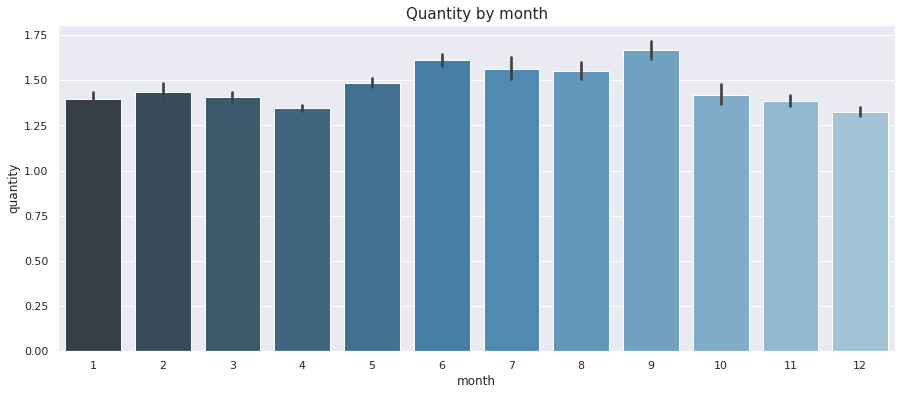Examining the revenue generated by month shows a similar picture but is better at showing how the value of orders change over the course of the year. Again the summer months are busier, with revenue peaking in September.

``````f, ax = plt.subplots(figsize=(15, 6))
ax = sns.barplot(x="month",
y="revenue",
data=df_12m,
palette="Blues_d")\
.set_title("Revenue by month",fontsize=15)
``````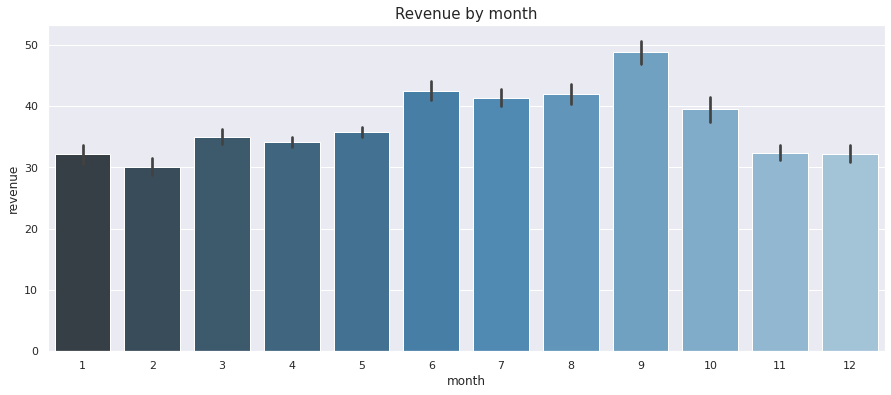Now we’ve taken a look at the data, we need to reformat it so we’ve got the monthly data for each SKU neatly partitioned in a Pandas dataframe. First, we’ll `groupby()` the `sku` and `month` column and create a dataframe based on the `sum()` of the `quantity` field.

``````df_12m_units = df_12m.groupby(['sku','month'])['quantity'].sum().to_frame().reset_index()
``````
sku month quantity
0 001TC 1 5
1 001TC 2 3
2 001TC 3 9
3 001TC 4 6
4 001TC 6 4

Since our above data is in long format, rather than wide format, we need to reshape the data using Pandas. We can do this using the `pivot()` function. We’ll put each SKU on its own line and store the quantity of units sold in each month in its own column.

``````df_12m_units = df_12m_units.pivot(index='sku', columns='month', values='quantity')\
``````
month sku m1 m2 m3 m4 m5 m6 m7 m8 m9 m10 m11 m12
0 001TC 5.0 3.0 9.0 6.0 0.0 4.0 5.0 2.0 6.0 0.0 4.0 0.0
1 003TC 11.0 6.0 12.0 29.0 25.0 19.0 5.0 8.0 3.0 0.0 5.0 0.0
2 005A 0.0 0.0 0.0 0.0 0.0 0.0 0.0 0.0 0.0 0.0 3.0 0.0
3 0068S 1.0 0.0 4.0 3.0 1.0 0.0 5.0 4.0 1.0 0.0 1.0 0.0
4 008APCS 6.0 3.0 12.0 18.0 38.0 5.0 15.0 15.0 5.0 0.0 3.0 11.0

#### Calculate standard deviation in demand

Next we need to calculate the standard deviation in the monthly demand for each SKU. We can do this by creating a subset of the month columns and appending `.std(axis=1)` in order to calculate the standard deviation in the values within each row. We’ll assign that value back to the dataframe in a new column.

``````df_12m_units['std_demand'] = df_12m_units[['m1','m2','m3','m4','m5','m6',
'm7','m8','m9','m10','m11','m12']].std(axis=1)
``````
``````df_12m_units.head()
``````
month sku m1 m2 m3 m4 m5 m6 m7 m8 m9 m10 m11 m12 std_demand
0 001TC 5.0 3.0 9.0 6.0 0.0 4.0 5.0 2.0 6.0 0.0 4.0 0.0 2.806918
1 003TC 11.0 6.0 12.0 29.0 25.0 19.0 5.0 8.0 3.0 0.0 5.0 0.0 9.488031
2 005A 0.0 0.0 0.0 0.0 0.0 0.0 0.0 0.0 0.0 0.0 3.0 0.0 0.866025
3 0068S 1.0 0.0 4.0 3.0 1.0 0.0 5.0 4.0 1.0 0.0 1.0 0.0 1.825742
4 008APCS 6.0 3.0 12.0 18.0 38.0 5.0 15.0 15.0 5.0 0.0 3.0 11.0 10.255449

#### Calculate total demand

Since we also need to know the annual demand, we next need to calculate the `sum()` of all of the monthly data, which we can do simply by adding the columns together and then assigning them to a new field using the `assign()` function.

``````df_12m_units = df_12m_units.assign(total_demand = df_12m_units['m1'] + df_12m_units['m2'] + \
df_12m_units['m3'] + df_12m_units['m4'] + \
df_12m_units['m5'] + df_12m_units['m6'] + \
df_12m_units['m7'] + df_12m_units['m8'] + \
df_12m_units['m9'] + df_12m_units['m10'] + \
df_12m_units['m11'] + df_12m_units['m12']
)
``````
``````df_12m_units.head()
``````
month sku m1 m2 m3 m4 m5 m6 m7 m8 m9 m10 m11 m12 std_demand total_demand
0 001TC 5.0 3.0 9.0 6.0 0.0 4.0 5.0 2.0 6.0 0.0 4.0 0.0 2.806918 44.0
1 003TC 11.0 6.0 12.0 29.0 25.0 19.0 5.0 8.0 3.0 0.0 5.0 0.0 9.488031 123.0
2 005A 0.0 0.0 0.0 0.0 0.0 0.0 0.0 0.0 0.0 0.0 3.0 0.0 0.866025 3.0
3 0068S 1.0 0.0 4.0 3.0 1.0 0.0 5.0 4.0 1.0 0.0 1.0 0.0 1.825742 20.0
4 008APCS 6.0 3.0 12.0 18.0 38.0 5.0 15.0 15.0 5.0 0.0 3.0 11.0 10.255449 131.0

#### Calculate average monthly demand

To calculate the average demand for each SKU across the year we can divide the `total_demand` column value by the number of months in the dataset - 12 - to obtain the mean for the period.

``````df_12m_units = df_12m_units.assign(avg_demand = df_12m_units['total_demand'] / 12 )
``````
``````df_12m_units.head()
``````
month sku m1 m2 m3 m4 m5 m6 m7 m8 m9 m10 m11 m12 std_demand total_demand avg_demand
0 001TC 5.0 3.0 9.0 6.0 0.0 4.0 5.0 2.0 6.0 0.0 4.0 0.0 2.806918 44.0 3.666667
1 003TC 11.0 6.0 12.0 29.0 25.0 19.0 5.0 8.0 3.0 0.0 5.0 0.0 9.488031 123.0 10.250000
2 005A 0.0 0.0 0.0 0.0 0.0 0.0 0.0 0.0 0.0 0.0 3.0 0.0 0.866025 3.0 0.250000
3 0068S 1.0 0.0 4.0 3.0 1.0 0.0 5.0 4.0 1.0 0.0 1.0 0.0 1.825742 20.0 1.666667
4 008APCS 6.0 3.0 12.0 18.0 38.0 5.0 15.0 15.0 5.0 0.0 3.0 11.0 10.255449 131.0 10.916667

#### Calculate Coefficient of Variation in demand

Finally, we can calculate the amount of variation seen in demand for each SKU across the year. To measure the variation, and therefore the forecastability, we can use a calculation called the Coefficient of Variation or CV. This is the mean demand over the standard deviation in demand. A value closer to zero implies that there’s minimal variation and higher predictability, while high values imply the opposite.

``````df_12m_units['cov_demand'] = df_12m_units['std_demand'] / df_12m_units['avg_demand']
``````

Once you’ve calculated the Coefficient of Variation in monthly product demand, it’s worth printing the `head()` and `tail()` of your dataframe to examine the most and least variable products in your inventory. You’ll notice that the set below all have a 3.46 value for their `cov_demand`, which is because they all sold in just one month in the 12 within the dataset.

``````df_12m_units.sort_values(by='cov_demand', ascending=False).head(5)
``````
month sku m1 m2 m3 m4 m5 m6 m7 m8 m9 m10 m11 m12 cov_demand
3892 SKU1 0.0 0.0 0.0 0.0 0.0 0.0 0.0 0.0 0.0 11.0 0.0 0.0 3.464102
5313 SKU2 0.0 0.0 0.0 0.0 0.0 0.0 0.0 0.0 19.0 0.0 0.0 0.0 3.464102
3404 SKU3 0.0 0.0 0.0 0.0 0.0 0.0 0.0 0.0 0.0 17.0 0.0 0.0 3.464102
4961 SKU4 0.0 0.0 0.0 0.0 0.0 0.0 0.0 0.0 0.0 0.0 19.0 0.0 3.464102
5356 SKU5 0.0 0.0 0.0 0.0 0.0 0.0 0.0 0.0 0.0 1.0 0.0 0.0 3.464102
``````df_12m_units.sort_values(by='cov_demand', ascending=True).head(5)
``````
month sku m1 m2 m3 m4 m5 m6 m7 m8 m9 m10 m11 m12 cov_demand
95 SKU100 19.0 28.0 29.0 35.0 35.0 37.0 33.0 29.0 26.0 18.0 17.0 24.0 0.251218
4135 SKU101 8.0 7.0 9.0 6.0 9.0 5.0 7.0 6.0 5.0 4.0 6.0 5.0 0.252679
2848 SKU102 37.0 32.0 28.0 35.0 33.0 20.0 30.0 22.0 12.0 33.0 33.0 27.0 0.254565
3925 SKU103 17.0 22.0 18.0 29.0 16.0 23.0 20.0 13.0 14.0 19.0 12.0 16.0 0.262395
2954 SKU104 10.0 7.0 5.0 9.0 8.0 9.0 5.0 6.0 6.0 5.0 5.0 7.0 0.263513

The `min()`, `mean()`, and `max()` values show that the lowest CV is 0.25, the highest is 3.46, and the overall mean is 1.54. That means this dataset includes lots of products with fluctuating or seasonal demand, which is going to make things much harder for procurement staff to keep in check.

``````df_12m_units['cov_demand'].min()
``````
``````0.25121791989373
``````
``````df_12m_units['cov_demand'].mean()
``````
``````1.5455514561139476
``````
``````df_12m_units['cov_demand'].max()
``````
``````3.464101615137755
``````

Here’s the spread of CV across the product range. Given that items with a CV over 1 are much harder to predict due to the fluctuations in demand, this particularly inventory could be a relatively challenging one to keep in good shape, unless the company held expensive levels of buffer inventory.

``````f, ax = plt.subplots(figsize=(15, 6))
ax = sns.distplot(df_12m_units['cov_demand']).set_title("Coefficient of Variation",fontsize=15)
``````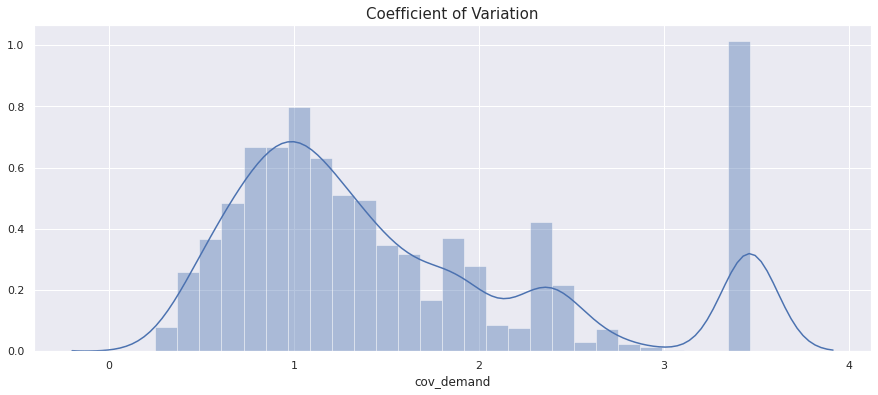#### Assign XYZ classes

As with ABC inventory analysis, you’re free to select your own parameters for the designation of SKUs into the XYZ classes. However, the most common approach is to use the Coefficient of Variation to guide you. Items with a CV of 0.5 or lower are very predictable and have stable sales all year round, so these go in Class X. Items with a CV of 0.5 to 1.0 are less predictable and harder to manage, so these go in Class Y. Finally, everything with a CV over 1 goes in Class Z, as it’s much harder to forecast and subject to fluctuations throughout the year, often because it only sells sporadically.

We’ll create a function to take the `cov_demand` value from our dataframe and then assign the relevant Class based on the Coefficient of Variation XYZ rules we defined above. If you use `value_counts()` you’ll get a quick summary of the numbers of SKUs in each class. As we can see from the above graph, we’ve only got 236 in the highly predictable Class X, plus 1512 in the less predictable Class Y, while we have a hard to manage 3609 in Class Z.

``````def xyz_classify_product(cov):
"""Apply an XYZ classification to each product based on
its coefficient of variation in order quantity.

:param cov: Coefficient of variation in order quantity for SKU
:return: XYZ inventory classification class
"""

if cov <= 0.5:
return 'X'
elif cov > 0.5 and cov <= 1.0:
return 'Y'
else:
return 'Z'
``````
``````df_12m_units['xyz_class'] = df_12m_units['cov_demand'].apply(xyz_classify_product)
df_12m_units.xyz_class.value_counts()
``````
``````Z    3609
Y    1512
X     236
Name: xyz_class, dtype: int64
``````

#### Examine the XYZ classes

To take a look at the distribution of the XYZ classes we can print out our dataframe and then run an aggregation to calculate some summary statistics. Most of the SKUs that are easy to predict have relatively low demand based on the number of units. It’s those in Classes Y and Z which sell in the highest volumes.

``````df_12m_units.head()
``````
month sku m1 m2 m3 m4 m5 m6 m7 m8 std_demand total_demand avg_demand cov_demand xyz_class
0 SKU1 5.0 3.0 9.0 6.0 0.0 4.0 5.0 2.0 2.806918 44.0 3.666667 0.765523 Y
1 SKU2 11.0 6.0 12.0 29.0 25.0 19.0 5.0 8.0 9.488031 123.0 10.250000 0.925662 Y
2 SKU3 0.0 0.0 0.0 0.0 0.0 0.0 0.0 0.0 0.866025 3.0 0.250000 3.464102 Z
3 SKU4 1.0 0.0 4.0 3.0 1.0 0.0 5.0 4.0 1.825742 20.0 1.666667 1.095445 Z
4 SKU6 6.0 3.0 12.0 18.0 38.0 5.0 15.0 15.0 10.255449 131.0 10.916667 0.939430 Y
``````df_12m_units.groupby('xyz_class').agg(
total_skus=('sku', 'nunique'),
total_demand=('total_demand', 'sum'),
std_demand=('std_demand', 'mean'),
avg_demand=('avg_demand', 'mean'),
avg_cov_demand=('cov_demand', 'mean'),
)
``````
total_skus total_demand std_demand avg_demand avg_cov_demand
xyz_class
X 236 30144.0 4.271093 10.644068 0.418543
Y 1512 166133.0 7.494467 9.156360 0.781056
Z 3609 144532.0 4.354399 3.337305 1.939536

#### Plot the XYZ data

To plot the XYZ data and understand how each of the Classes changes in demand across the year, we need to reshape our data again. We’ll create a new dataframe called `df_monthly` and will use a `groupby()` function to group products by their `xyz_class` and then sum the revenue by month.

``````df_monthly = df_12m_units.groupby('xyz_class').agg(
m1=('m1', 'sum'),
m2=('m2', 'sum'),
m3=('m3', 'sum'),
m4=('m4', 'sum'),
m5=('m5', 'sum'),
m6=('m6', 'sum'),
m7=('m7', 'sum'),
m8=('m8', 'sum'),
m9=('m9', 'sum'),
m10=('m10', 'sum'),
m11=('m11', 'sum'),
m12=('m12', 'sum'),
)

``````
m1 m2 m3 m4 m5 m6 m7 m8 m9 m10 m11 m12
xyz_class
X 2495.0 2388.0 2718.0 2922.0 3125.0 2777.0 2402.0 2432.0 2153.0 1993.0 2470.0 2269.0
Y 6218.0 6886.0 12582.0 23269.0 26799.0 20835.0 16899.0 18408.0 17052.0 6024.0 6039.0 5122.0
Z 2929.0 3241.0 8897.0 24075.0 32435.0 20367.0 14650.0 14836.0 13270.0 4108.0 3331.0 2393.0

For plotting, we need to convert the data from wide format to long format, so we’ll use the `unstack()` function to create a long dataframe, then we’ll reset the index and rename the columns to match the underlying data.

``````df_monthly_unstacked = df_monthly.unstack('xyz_class').to_frame()
df_monthly_unstacked = df_monthly_unstacked.reset_index().rename(columns={'level_0': 'month', 0: 'demand'})
``````
month xyz_class demand
0 m1 X 2495.0
1 m1 Y 6218.0
2 m1 Z 2929.0
3 m2 X 2388.0
4 m2 Y 6886.0

Plotting the Class X data shows that the demand for these products is relatively steady throughout the year. In less seasonal businesses where the SKUs sell at similar rates all year, we’d expect to see almost no variation, but the changes here show that this retailer does have more seasonal SKUs than most.

``````f, ax = plt.subplots(figsize=(15, 6))
ax = sns.barplot(x="month",
y="demand",
data=df_monthly_unstacked[df_monthly_unstacked['xyz_class']=='X'],
palette="Blues_d")\
.set_title("X class demand by month",fontsize=15)
``````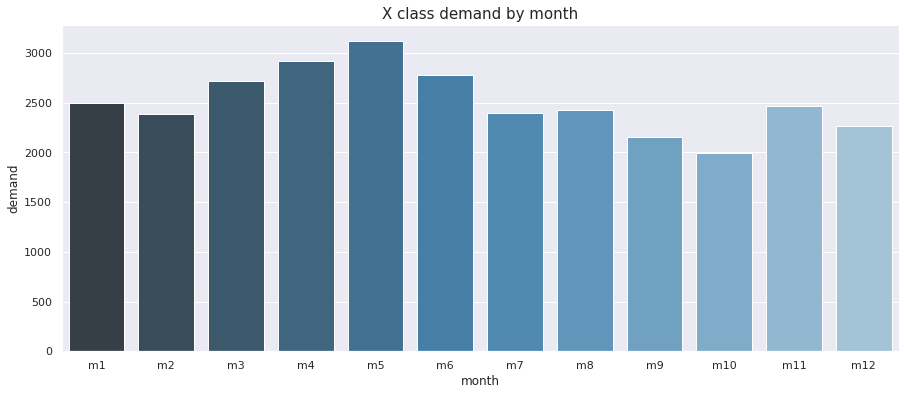The Class Y data shows why these SKUs are harder to control. The warmer months see some big changes in demand and then sales of these SKUs drop away significantly as soon as autumn arrives.

``````f, ax = plt.subplots(figsize=(15, 6))
ax = sns.barplot(x="month",
y="demand",
data=df_monthly_unstacked[df_monthly_unstacked['xyz_class']=='Y'],
palette="Blues_d")\
.set_title("Y class demand by month",fontsize=15)
``````The Class Z SKUs are the least predictable of all. They’re relatively similar in pattern to Class Y, but the impact is slightly more dramatic because some of the SKUs only sell during the summer and some only sell once per year.

``````f, ax = plt.subplots(figsize=(15, 6))
ax = sns.barplot(x="month",
y="demand",
data=df_monthly_unstacked[df_monthly_unstacked['xyz_class']=='Z'],
palette="Blues_d")\
.set_title("Z class demand by month",fontsize=15)
``````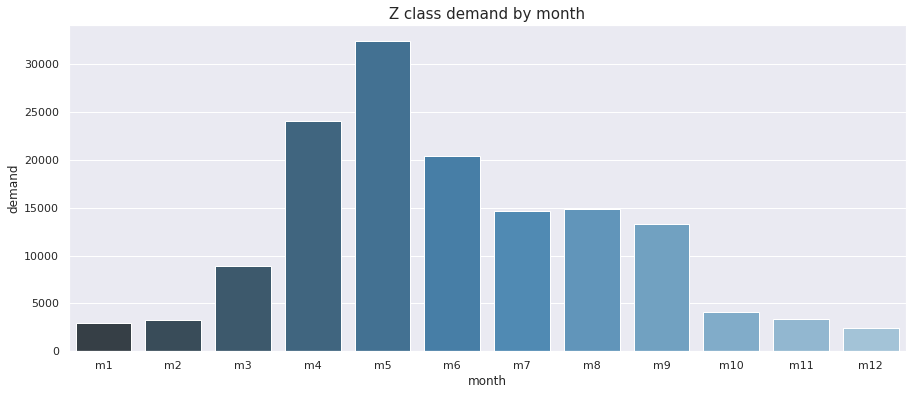Here they all are side by side. The really interesting thing here, when they’re all shown on the same scale, is that not only are the hard to predict Class Y and Class Z SKUs seasonal over the summer months, the spike in demand they generate is massive.

``````f, ax = plt.subplots(figsize=(15, 6))
ax = sns.barplot(x="month",
y="demand",
hue="xyz_class",
data=df_monthly_unstacked,
palette="Blues_d")\
.set_title("XYZ demand by month",fontsize=15)
``````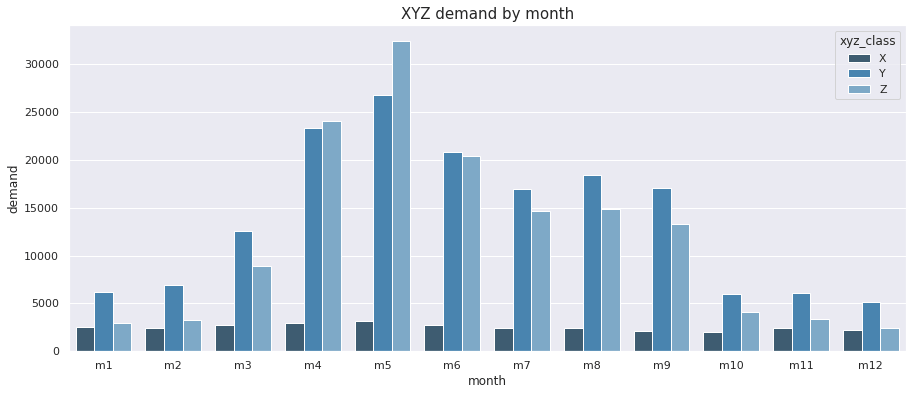#### ABC inventory analysis

Since XYZ analysis only looks at the unit volume demand, we next need to perform an ABC inventory analysis to determine the revenue impact each provides. We’ll re-use the 12 month dataframe we created earlier and will then run an aggregation to calculate the revenue metrics for each SKU.

``````df_skus = df_12m.groupby('sku').agg(
unique_purchases=('sku', 'nunique'),
total_units=('quantity', 'sum'),
total_revenue=('revenue', 'sum'),
).sort_values(by='total_revenue', ascending=False).reset_index()
``````
``````df_skus.head()
``````
sku unique_purchases total_units total_revenue
0 SKU1 1 2526 125478.21
1 SKU2 1 1059 105242.81
2 SKU3 1 2107 91694.40
3 SKU4 1 562 67302.45
4 SKU5 1 707 60823.65

To calculate ABC classes we need to know the total revenue generated by all SKUs and the cumulative percentage revenue contribution generated by each SKU. It’s vital that they’re sorted in descending order of the total revenue they generated in order for this to work, as we did above. We can use the `cumsum()` function to calculate the cumulative sum of revenue and then calculate the running percentage of revenue and store it in the dataframe.

``````df_skus['revenue_cumsum'] = df_skus['total_revenue'].cumsum()
df_skus['revenue_total'] = df_skus['total_revenue'].sum()
df_skus['revenue_running_percentage'] = (df_skus['revenue_cumsum'] / df_skus['revenue_total']) * 100
``````
``````df_skus.head()
``````
sku unique_purchases total_units total_revenue revenue_cumsum revenue_total revenue_running_percentage
0 SKU1 1 2526 125478.21 125478.21 8731132.47 1.437136
1 SKU2 1 1059 105242.81 230721.02 8731132.47 2.642510
2 SKU3 1 2107 91694.40 322415.42 8731132.47 3.692710
3 SKU4 1 562 67302.45 389717.87 8731132.47 4.463543
4 SKU5 1 707 60823.65 450541.52 8731132.47 5.160173

As with the XYZ classification, we’ll create a function to assign each product to the relevant Class based on its contribution to the percentage revenue. You can use whatever split best suits your data. I’ve assigned the SKUs that generate the top 80% of the revenue to Class A, the next 10% of revenue generators to Class B, and the rest to Class C.

``````def abc_classify_product(percentage):
"""Apply an ABC classification to each product based on
its ranked percentage revenue contribution. Any split
can be used to suit your data.

:param percentage: Running percentage of revenue contributed
:return: ABC inventory classification
"""

if percentage > 0 and percentage <= 80:
return 'A'
elif percentage > 80 and percentage <= 90:
return 'B'
else:
return 'C'
``````

Next, apply the `abc_classify_product()` function above and assign the value to the dataframe. It’s also useful to calculate the product sales rank at the same time, which you can do using the `rank()` function.

``````df_skus['abc_class'] = df_skus['revenue_running_percentage'].apply(abc_classify_product)
df_skus['abc_rank'] = df_skus['revenue_running_percentage'].rank().astype(int)
``````
sku total_revenue revenue_cumsum revenue_total revenue_running_percentage abc_class abc_rank
0 SKU1 125478.21 125478.21 8731132.47 1.437136 A 1
1 SKU2 105242.81 230721.02 8731132.47 2.642510 A 2
2 SKU3 91694.40 322415.42 8731132.47 3.692710 A 3
3 SKU4 67302.45 389717.87 8731132.47 4.463543 A 4
4 SKU5 60823.65 450541.52 8731132.47 5.160173 A 5

If we now examine the stats for each of the Classes, we see that we’ve got 1171 in Class A generating 80% of our revenue. There are 738 in Class B, generating the next 10% and then a massive 3448 in Class C, which generate the bottom 10% of sales. Clearly, some management effort could be saved by focusing mostly on the A and B SKUs, instead of getting bogged down on the much slower selling Class C SKUs.

``````df_abc = df_skus.groupby('abc_class').agg(
total_skus=('sku', 'nunique'),
total_units=('total_units', sum),
total_revenue=('total_revenue', sum),
).reset_index()

df_abc
``````
abc_class total_skus total_units total_revenue
0 A 1171 208560 6983670.13
1 B 738 44042 873627.71
2 C 3448 88207 873834.63

Here’s how the ABC Class data looks in terms of the revenue generated per class - you can see the 80/10/10 split we used.

``````f, ax = plt.subplots(figsize=(15, 6))
ax = sns.barplot(x="abc_class",
y="total_revenue",
data=df_abc,
palette="Blues_d")\
.set_title("Revenue by ABC class",fontsize=15)
``````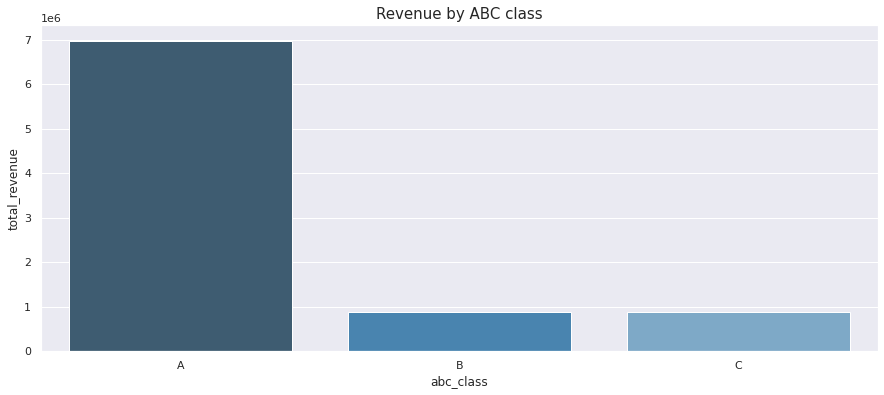As you can see, there’s a shedload of SKUs in Class C, which only generate 10% of the company’s total revenue.

``````f, ax = plt.subplots(figsize=(15, 6))
ax = sns.barplot(x="abc_class",
y="total_skus",
data=df_abc,
palette="Blues_d")\
.set_title("SKUs by ABC class",fontsize=15)
``````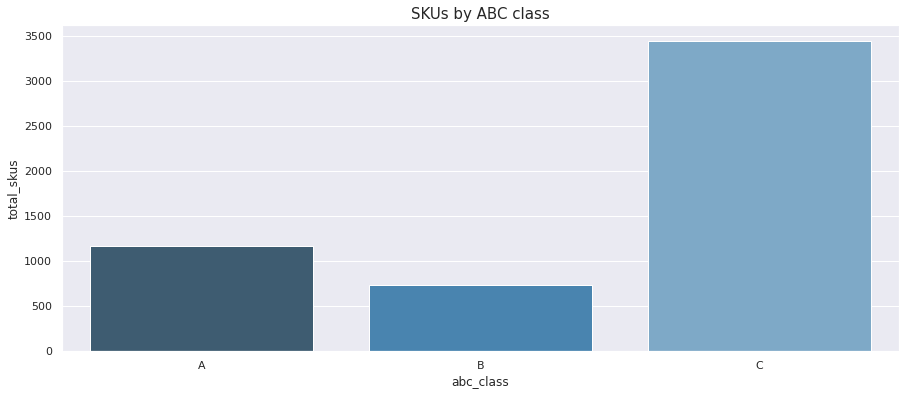In terms of the quantities of units sold, there are obviously lots in Class A, as they made up 80% of the revenue, but there are also loads in Class C, which suggests there are lots of cheap, seasonal items in here, or things that only sell sporadically.

``````f, ax = plt.subplots(figsize=(15, 6))
ax = sns.barplot(x="abc_class",
y="total_units",
data=df_abc,
palette="Blues_d")\
.set_title("Quantity by ABC class",fontsize=15)
``````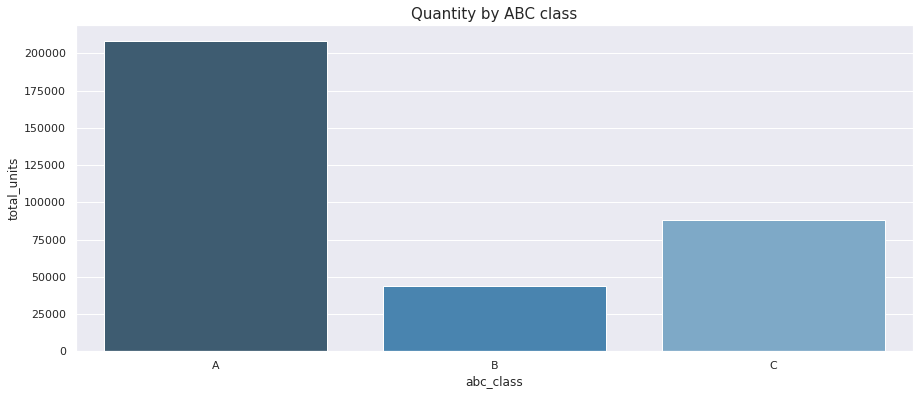## ABC-XYZ inventory analysis

The final step is to bring together our XYZ inventory management data with our ABC inventory management data. We’ll select the key metrics we want to keep from our ABC analysis and create a new dataframe, then we’ll join that to the XYZ analysis data.

``````df_abc = df_skus[['sku','abc_class','abc_rank','total_revenue']]
df_xyz = df_12m_units.copy()
df_abc_xyz = df_abc.merge(df_xyz, on='sku', how='left')
``````
sku abc_class abc_rank total_revenue std_demand total_demand avg_demand cov_demand xyz_class
0 SKU1 A 1 125478.21 180.563512 2526.0 210.500000 0.857784 Y
1 SKU2 A 2 105242.81 78.563379 1059.0 88.250000 0.890237 Y
2 SKU3 A 3 91694.40 143.161357 2107.0 175.583333 0.815347 Y
3 SKU4 A 4 67302.45 32.078975 562.0 46.833333 0.684960 Y
4 SKU5 A 5 60823.65 49.522187 707.0 58.916667 0.840546 Y

5 rows × 21 columns

#### Creating the ABC-XYZ class

Now we’ve got the data all in one place, we can create the ABC-XYZ class designations by concatenating the string value of the `abc_class` to the `xyz_class`. We’ll then create a new dataframe of summary statistics by grouping on the new `abc_xyz_class` and calculating the number of SKUs, the demand volume, the average demand, and the total revenue by class.

``````df_abc_xyz['abc_xyz_class'] = df_abc_xyz['abc_class'].astype(str) + df_abc_xyz['xyz_class'].astype(str)
``````

Most of our revenue comes from products in Class AZ. These contribute the top 80% of the revenue but are also seasonal and harder to predict. At the bottom, we’ve got a small group of Class C SKUs which contribute little, but on the plus side are not very variable, so are easier to predict.

``````df_abc_xyz_summary = df_abc_xyz.groupby('abc_xyz_class').agg(
total_skus=('sku', 'nunique'),
total_demand=('total_demand', sum),
avg_demand=('avg_demand', 'mean'),
total_revenue=('total_revenue', sum),
).reset_index()

df_abc_xyz_summary.sort_values(by='total_revenue', ascending=False)
``````
abc_xyz_class total_skus total_demand avg_demand total_revenue
2 AZ 709 92142.0 10.830042 3597378.39
1 AY 371 100443.0 22.561321 3016842.12
5 BZ 470 17313.0 3.069681 559901.76
8 CZ 2430 35077.0 1.202915 532094.09
0 AX 91 15975.0 14.629121 369449.62
7 CY 925 45976.0 4.141982 298143.90
4 BY 216 19714.0 7.605710 253923.36
3 BX 52 7015.0 11.241987 59802.59
6 CX 93 7154.0 6.410394 43596.64
``````f, ax = plt.subplots(figsize=(15, 6))
ax = sns.barplot(x="abc_xyz_class",
y="total_skus",
data=df_abc_xyz_summary,
palette="Blues_d")\
.set_title("SKUs by ABC-XYZ class",fontsize=15)
``````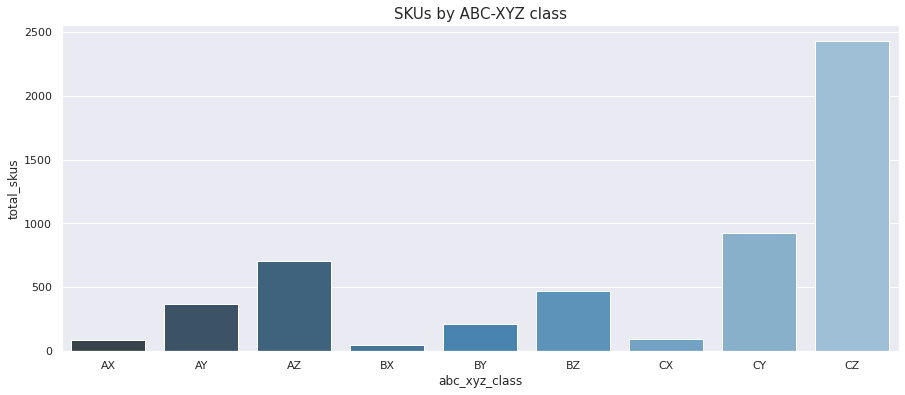``````f, ax = plt.subplots(figsize=(15, 6))
ax = sns.barplot(x="abc_xyz_class",
y="total_revenue",
data=df_abc_xyz_summary,
palette="Blues_d")\
.set_title("Revenue by ABC-XYZ class",fontsize=15)
``````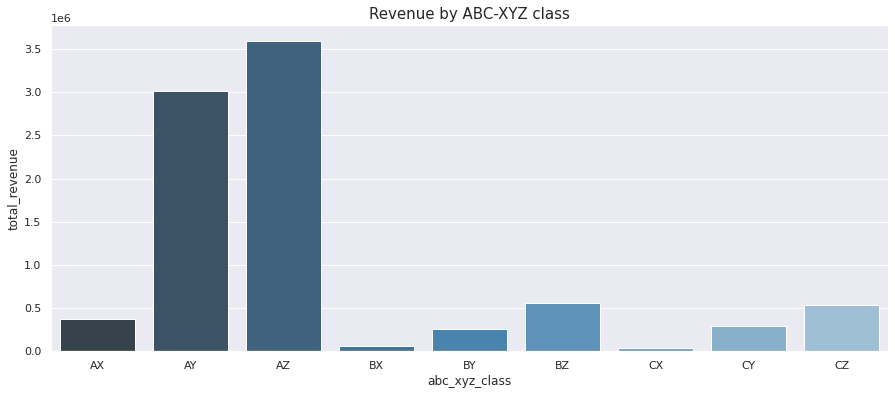``````f, ax = plt.subplots(figsize=(15, 6))
ax = sns.barplot(x="abc_xyz_class",
y="total_demand",
data=df_abc_xyz_summary,
palette="Blues_d")\
.set_title("Demand by ABC-XYZ class",fontsize=15)
``````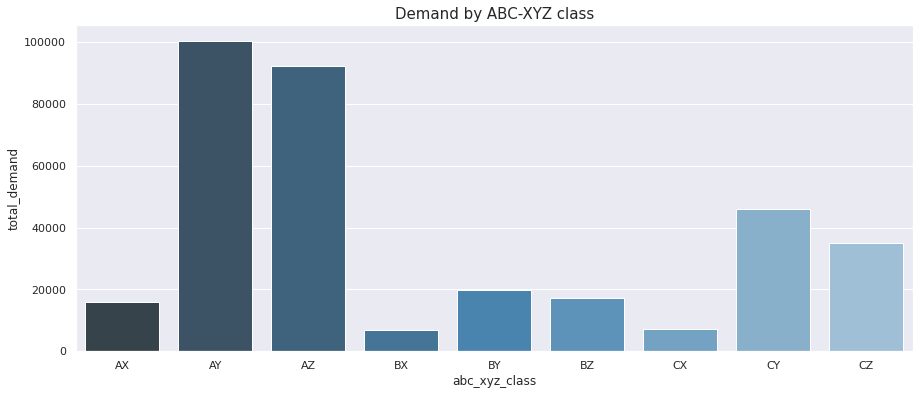#### Understanding the ABC XYZ classes

By combining the ABC and XYZ data we generate nine distinct classes. The Classes with an A prefix all contribute high amounts of revenue, the B Classes contribute medium revenue and the C Classes contribute low revenue.

Similarly, the X suffixed Classes have steady demand and are easy to forecast and manage. The Y suffixed Classes have variable but predictable demand so are harder to manage than the X Classes, but still easily doable. The Z Classes are the toughest of all. They’re sold sporadically and in varying volumes, so they’re harder to forecast and harder to manage.

Chances are, your operations and procurement team will already understand how to apply ABC XYZ inventory classification, given that it’s the industry standard and is included within major platforms such as SAP.

 AX High value Steady demand Easy to forecast Easy to manage BX Medium value Steady demand Easy to forecast Easy to manage CX Low value Steady demand Easy to forecast Easy to manage AY High value Variable demand Harder to forecast Harder to manage BY Medium value Variable demand Harder to forecast Harder to manage CY Low value Variable demand Harder to forecast Harder to manage AZ High value Sporadic demand Difficult to forecast Difficult to manage BZ Medium value Sporadic demand Difficult to forecast Difficult to manage CZ Low value Sporadic demand Difficult to forecast Difficult to manage

#### Management approaches

The simplest solution to avoiding stock-outs is to simply hold more stock by increasing your buffer inventory levels. This has many advantages: You’ll lose fewer sales, so AOVs and revenue will be higher; you’ll likely see better customer retention and higher levels of satisfaction because customers don’t go elsewhere when they see an item is out of stock, and you won’t need to procure expensive emergency replenishment stock and erode your margin.

However, holding higher stocks also has some disadvantages: You’ll need to spend more on stock; you’ll have more capital tied up in stock; you’ll need more space, equipment, and staff to handle the stock; insurance costs will go up with your stock valuation. What’s needed, therefore, is a compromise which sits somewhere in the middle.

The Association of International Certified Professional Accountants has some very good information on the practical application of ABC XYZ classifications for procurement managers to follow to maximise the revenue and profit for the business, without tying up loads of capital in stock. It recommends the following approach:

 AX Automatic replenishment Low buffer, JIT, or consignment transfers Perpetual inventory BX Automatic replenishment Periodic counting Low buffer CX Automatic replenishment Periodic estimation Low buffer AY Semi-automatic replenishment Low buffer BY Semi-automatic replenishment Manually adjusted seasonal buffer CY Semi-automatic replenishment High buffer AZ Buy to order No buffer Not stocked BZ Buy to order No buffer Lead time shown Not stocked CZ Automatic replenishment High buffer Periodic inspection

ABC XYZ inventory management is just one of a wide range of commonly used inventory control systems in operations management (including HML, VED, SDF, SOS, GOLF, and FNS), each of which sets out to solve some of the specific issues that can arise with different types of inventory, including the impact of a stock out on production. You can find out more about it in the papers below.